# Algebra 1 : How to find the number of integers between two other integers

## Example Questions

### Example Question #1 : How To Find The Number Of Integers Between Two Other Integers

Solve the expression using the given values below.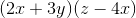,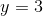,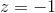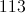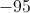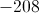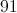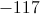Explanation:,,We can simply plug in the values to each occurrence of the variables.

For: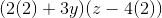Then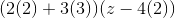Then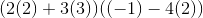Now we can solve.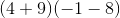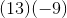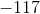### Example Question #1 : How To Find The Number Of Integers Between Two Other Integers

What is the sum of the prime integers that satisfy the inequality?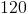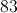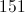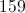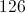Explanation:

The prime integers beterrn 20 and 40 are 23, 29, 31, and 37. Their sum is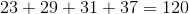.

### Example Question #1 : How To Find The Number Of Integers Between Two Other Integers

What is the sum of all of the perfect squares and perfect cubes that satisfy the inequality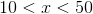?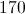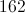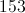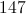Explanation:

There are four perfect squares between 10 and 50: 16, 25, 36, and 49. There is one perfect cube, 27, in this range. Add: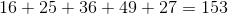### Example Question #1 : How To Find The Number Of Integers Between Two Other Integers

How many integers fall between 17 and 73 on a number line?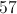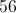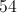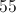Explanation:

To calculate the number of integers, find subtract the integers of interest and then subtract 1.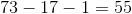As a proof of concept, calculate the number of integers that fall between 5 and 10 on a number line. We know there are 4 (6, 7, 8, 9).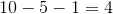### Example Question #2 : How To Find The Number Of Integers Between Two Other Integers

How many perfect squares satisfy the inequality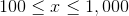?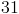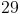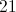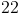Explanation:

The smallest perfect square between 100 and 1,000 inclusive is 100 itself, since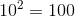. The largest can be found by noting that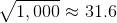; this makes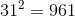the greatest perfect square in this range.

Since the squares of the integers from 10 to 31 all fall in this range, this makes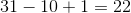perfect squares.

### Example Question #1 : How To Find The Number Of Integers Between Two Other Integers

An ice cream vendor sells five different flavors of ice cream.

In how many ways can you choose three scoops of different ice cream flavors if order matters?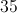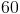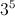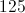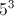Explanation:

There are five ways to choose the first scoop, then four ways to choose the second scoop, and finally three ways to choose the third scoop:

5 * 4 * 3 = 60

### Example Question #1 : How To Find The Number Of Integers Between Two Other Integers

How many integers are in between the numbers 455, and 467?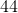Explanation:

How many integers are in between the numbers 455, and 467?

An integer is just a whole number.

We can find the number of integers between two numbers in a variety of ways. To start, let's count off.

456,457,458,459,460,461,462,463,464,465,466

There are 11.

However, what if your numbers are really big?

Then just subtract the smaller number from the bigger number and then subtract 1.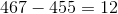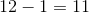Our answer is eleven either way.

### Example Question #1 : How To Find The Number Of Integers Between Two Other Integers

Find how many integers are between two and eight.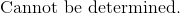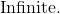Explanation:

To determine the number of integers in between 2 and 8, we can simply write out the numbers and count.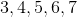Notice that the number of integers in between two other integers will be one less than the range of the two given numbers.

The answer is: